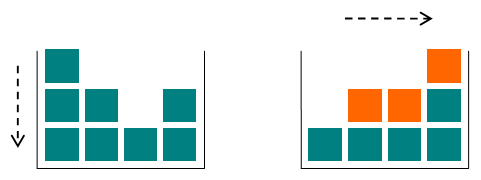# CF405A Gravity Flip

• 190通过
• 270提交
• 题目来源
• 评测方式 RemoteJudge
• 标签 快速排序,快排 排序 桶排
• 难度 入门难度
• 时空限制 1000ms / 256MB
• 提示：收藏到任务计划后，可在首页查看。

## 题意翻译

### 题目描述:

小克里斯在物理课上很无聊（太容易了），所以他建了一个玩具箱让自己的有事做。这个盒子很特别，因为它有改变重力的能力。 盒子内排列 NN 列的玩具立方体。第$i$列包含$A_i$个立方体。首先，盒子里的重力向下拉动立方体。当克里斯切换重力时，它开始将所有立方体拉到盒子的右侧。该图显示了方块中的立方体的初始和最终位置：改变了它们的位置的立方体用橙色突出显示。 给定盒子中的玩具立方体的初始位置，在重力开关之后找到$N$列中的每个立方体的数量！

### 输入格式：

输入的第一行包含整数$n(1<=n<=100)$，表示玩具箱中玩具的列数。下一行包含 nn 个用空隔分隔的整数。第$i$个数$A_i(1<=A_i<=100)$表示第i列中立方体的数目。

### 输出格式：

输出$n$个整数，由空隔分隔，其中第$i$个数是重力切换后第$i$列中的立方体数量。

## 题目描述

Little Chris is bored during his physics lessons (too easy), so he has built a toy box to keep himself occupied. The box is special, since it has the ability to change gravity.

There are $n$ columns of toy cubes in the box arranged in a line. The $i$ -th column contains $a_{i}$ cubes. At first, the gravity in the box is pulling the cubes downwards. When Chris switches the gravity, it begins to pull all the cubes to the right side of the box. The figure shows the initial and final configurations of the cubes in the box: the cubes that have changed their position are highlighted with orange.Given the initial configuration of the toy cubes in the box, find the amounts of cubes in each of the $n$ columns after the gravity switch!

## 输入输出格式

输入格式：

The first line of input contains an integer $n$ ( $1<=n<=100$ ), the number of the columns in the box. The next line contains $n$ space-separated integer numbers. The $i$ -th number $a_{i}$ ( $1<=a_{i}<=100$ ) denotes the number of cubes in the $i$ -th column.

输出格式：

Output $n$ integer numbers separated by spaces, where the $i$ -th number is the amount of cubes in the $i$ -th column after the gravity switch.

## 输入输出样例

输入样例#1： 复制
4
3 2 1 2

输出样例#1： 复制
1 2 2 3

输入样例#2： 复制
3
2 3 8

输出样例#2： 复制
2 3 8


## 说明

The first example case is shown on the figure. The top cube of the first column falls to the top of the last column; the top cube of the second column falls to the top of the third column; the middle cube of the first column falls to the top of the second column.

In the second example case the gravity switch does not change the heights of the columns.

提示
标程仅供做题后或实在无思路时参考。
请自觉、自律地使用该功能并请对自己的学习负责。
如果发现恶意抄袭标程，将按照I类违反进行处理。